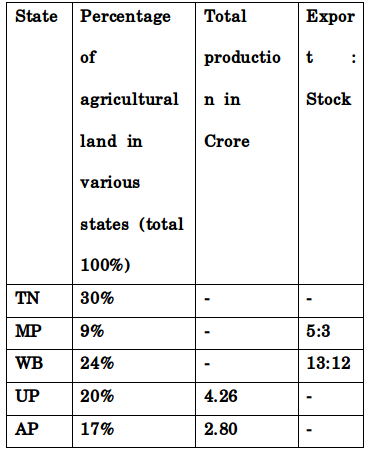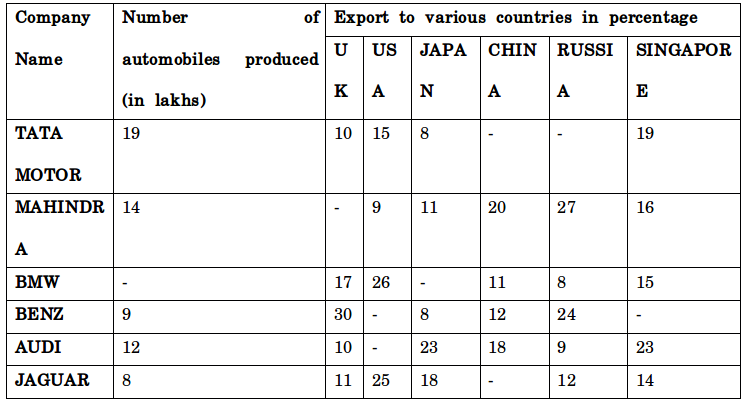## Important Data Interpretation For SBI PO : Set – 64

D.1-5) Study the following information carefully and answer the question given below.

Based on the below detail answer the given questions.Total production = Total land* Production per acre

Export = Total Production – Stock

1) In UP production per acre is 71,000 which is 0.4% of export in MP. Find the total production in MP.

a) 1.77 Cr

b) 1.06 Cr

c) 2.84 Cr

d) 3.01 Cr

e) None of these.

C)

Total production in UP = Total land in UP ×Production per acre

42600000 = Total land in UP × 71000

Total land in UP = 600 Acres = 20%

Production per acre in UP = 71000 = 0.4% of export in MP

Export in MP = 71000/0.4×100 = 1, 77, 50,000

In Mp ratio of Export to Stock is 5: 3

Therefore Stock in MP = 17750000/5×3 = 1,06,50,000

Total Production in MP = Export + Stock = 17750000 + 10650000 = 2, 84,00000 = 2.84 Cr.

2) Due to drought in every acre 30% of production was affected as originally expected in AP which is equal to production in 240 Acres of land. Due to this stock was reduced by 12% of originally planned value which is one-third of export. Export production is equal to production in how many acres land approximately?

a) 167

b) 160

c) 169

d) 163

e) None of these.

d)

Total production = 28000000 = 70% of originally expected

30% of affected production = 28000000/70×30 = 12000000

12000000 = Production in 240 Acres

Production in 1 acre = 50000

Let total export be X

Then, 1/3 × X = 12 % of Stock => 88% of stock = 1/3× X × 1/12 × 88 = Total stock

Export = Total production – Stock

X = 28000000 – 1/3× X × 1/12 × 88

X + 22X/9 = 28000000

Total export, X = 28000000 × 9/31

Export production is equal to production in,

= (28000000×9)/(31×500000) = 162.58 Acres = 163 (Approx)

3) Total production in WB is Two-Third production of UP then total quantity of export in WB & UP is equal. Stock in WB is what percentage of stock in UP?

a) 40.9 %

b) 43.89%

c) 48.9%

d) 50%

e) %9%

C)

Total production of WB = 2/3 ×42600000 = 28400000

Ratio of export to stock in WB is 13: 12

Stock in WB = 12/25 × 28400000 = 1,36,32,000

Therefore export in WB = 13/25 × 28400000 = 1,47,68,000 = Export in UP

Stock in UP = Total production – Export = 42600000 – 14768000 = 2,78,32,000

Percentage of stock in WB when compared to UP is,

= 13632000/27832000 × 100 = 48.9 %

4) TN has a total production of 4.5cr in 7500 Acres. Then what is the average production per acre in TN, UP & AP? Find the value approximately

a) 7000

b) 7063

c) 7058

d) 7036

e) 6000

Production per Acre in TN = 45000000/7500 = 6000

7500 Acre in TN is 30% of total agricultural land.

Total agricultural land in UP = 7500/30×20 = 5000 acres

Production per acre in UP = 42600000/5000 = 8520

Total agricultural land in AP = 7500/30×17 = 4250 Acres

Production per acre in AP = 28000000/4250 = 6588 (Approx)

Average production per acre in TN, UP & AP is,

= (6000+8520+6588)/3 = 7036

5) If total production in all the states is 16Cr then what is the ratio between total production in TN, MP & WB to UP & AP.

a) 440 : 351

b) 353 : 447

c) 441 : 329

d) 657 : 567

e) 447 : 353

e)

Total production in UP & AP = 4.26 + 2.80 = 7.06 Cr

Total production in TN, MP and WB = 16 – 7.06 = 8.94 Cr

Ratio between total productions in TN, MP and WB to UP & AP is, 894: 706

447: 353

D.6-10) The below table shows that the production of automobiles by different companies and corresponding exports to various countries.

The total production of automobiles by all companies is 84 Lakhs.6) Jaguar exported to china is what percentage of Mahindra exported to UK?

a) 60.22 %

b) 76.99%

c) 67.22%

d) 47.98%

e) None of these

C)

Percentage of jaguar exported to china = 100-(11+25+18+12+14) = 20 %

Total jaguar exported to china = 800000/100 × 20 = 160000

Percentage of Mahindra exported to UK = 100 – (9+11+20+27+16) = 17%

Total Mahindra exported to UK = 1400000/100 × 17 = 238000

Percentage of jaguar exported to china when compared to Mahindra exported to UK,

=160000/238000×100 = 67.22 %

7) Find the total number of automobiles exported to Russia. If total number of jaguar exported to china equals to the total number Tata motors exported to china.

a) 1865900

b) 1736400

c) 1689500

d) 1726400

e) None of these

d)

Total number jaguar exported to china = 160000 (From Q. 46) = Total number of Tata motors exported to china.

Percentage of Tata motors exported to china = 160000/1900000 × 100 = 8.4 %

Percentage of Tata motors exported to Russia = 100 – (10+15+8+8.4+19) = 39.6 %

Total number of Tata motors exported to Russia = 1900000/100 × 39.6 = 752400

Total number of Mahindra exported to Russia = 1400000/100 ×27 = 378000

Total number of BMW exported to Russia = 2200000/100 × 8 = 176000

Total number of BENZ exported to Russia = 900000/100 × 24 =216000

Total number of Audi exported to Russia = 1200000/100 ×9 = 108000

Total number of Jaguar exported to Russia = 800000/100 × 12 = 96000

Total number of automobiles exported to Russia = 752400+378000+176000+216000+108000+96000

= 17,26,400

8) Find the average numbers of BMW, Audi & Jaguar exported to Singapore?

a) 239000

b) 339333

c) 239333

d) 439333

e) None of these

c)

Total number of BMW exported to Singapore = 2200000/100 × 15 = 330000

Total number of Audi exported to Singapore = 1200000/100 × 23 = 276000

Total number of Jaguar exported to Singapore = 800000/100 × 14 = 112000

Average = (330000+276000+112000)/3 = 239333 (Approximately)

9) Find the ratio between automobiles exported to UK and Japan?

a) 160 : 161

b) 163 : 160

c) 167 : 165

d) 160 : 163

e) None of these

d)

Total number Automobiles exported to UK is,

= 1900000/100×10 + 1400000/100×17 + 2200000/100×17 + 900000/100×30 + 1200000/100×10 + 800000/100×11

=190000 + 238000 + 374000 + 270000 + 120000 + 88000 = 1280000

Total number Automobiles exported to Japan is,

= (1900/000)/100×8 + 1400000/100×11 + 2200000/100×23 + 900000/100×8 + 1200000/100×23 + 800000/100×18

=152000 + 154000 + 506000 + 72000 + 276000 + 144000 = 1304000

Ratio of automobiles exported to UK to Japan = 1280000: 1304000

160: 163

10) Tata motors exported to USA is what percentage of Jaguar exported to the same country?

a) 140.25%

b) 142.5%

c) 147.65%

d) 145.5%

e) None of these